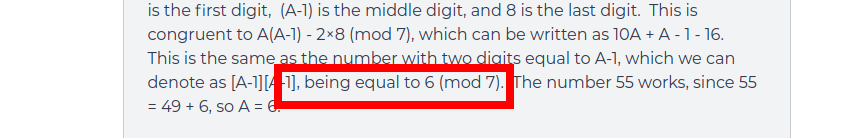# Question

• Part of the Solution:
$$\overline{AA4321} \equiv \overline{A[A-1]8} \equiv A(A-1)-2 \times 8 \equiv 10A+A-1-16 \pmod{7}.$$ This is the same as the number with two digits equal to A-1, which we can denote as $$\overline{[A-1][A-1]}.$$

Why is $$\overline{[A-1][A-1]}=10A+A-1-16$$ Isn't $$\overline{[A-1][A-1]}=10(A-1)+(A-1)=11A-11 \neq 10A+A-1-16?$$Instead of glossing over the solution, you are taking the time to go through everything thoroughly!

The solution is actually correct, though it skips a few steps, which might be the source of the confusion. Actually, the solution isn't exactly claiming that

$$\overline{AA4321} \equiv \overline{A[A-1]8} \equiv A(A-1)-2 \times 8 \equiv 10A+A-1-16 \pmod{7}$$ is the same as the number with two digits equal to A-1, which we can denote as $$\overline{[A-1][A-1]}.$$

It is really saying that

$$10A+A-1-16 \pmod{7} \equiv \overline{[A-1][A-1]} \text{ } (\textcolor{red}{6 \mod 7})$$This is because

\begin{aligned} 10A+A-1-16 \pmod{7} &= 11A - 17 \pmod{7} \\ & \equiv \textcolor{red}{[A-1][A-1] + 11} - 17 \pmod{7} \\ \end{aligned}

Why did we do this? It was a clever move, because $$[A][A]$$ and $$[A-1][A-1]$$ are both double-digit numbers. So it makes no difference to us if we are searching for a number of the form $$[A][A]$$ or $$[A-1][A-1]$$ -- we just think to ourselves, "I want to consider numbers of the form $$11, 22, 33, 44, 55, \ldots$$" but, conveniently, removing an $$11$$ from the $$[A][A]$$ allows us to cancel out some of the $$-17.$$

\begin{aligned} [A-1][A-1] \textcolor{red}{+ 11} - 17 \pmod{7} & \equiv [A-1][A-1] \textcolor{red}{- 6} \pmod{7} \\ \end{aligned}

This is congruent to $$0 \pmod{7}$$ when $$[A-1][A-1]$$ is congruent to $$6 \pmod{7},$$ and we see that when $$\boxed{A=6},$$ this works, since $$[6-1][6-1] = 55 \equiv 49 + 6 \pmod{7} \equiv 6 \pmod{7}.$$